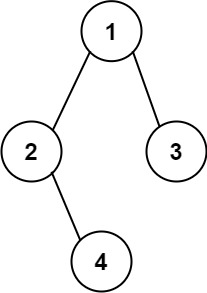# 606. Construct String from Binary Tree

Easy
Given the `root` of a binary tree, construct a string consisting of parenthesis and integers from a binary tree with the preorder traversal way, and return it.
Omit all the empty parenthesis pairs that do not affect the one-to-one mapping relationship between the string and the original binary tree.
Example 1:Input: root = [1,2,3,4]
Output:
"1(2(4))(3)"
Explanation:
Originally, it needs to be "1(2(4)())(3()())", but you need to omit all the unnecessary empty parenthesis pairs. And it will be "1(2(4))(3)"
Example 2:Input: root = [1,2,3,null,4]
Output:
"1(2()(4))(3)"
Explanation:
Almost the same as the first example, except we cannot omit the first parenthesis pair to break the one-to-one mapping relationship between the input and the output.
Constraints:
• The number of nodes in the tree is in the range `[1, 104]`.
• `-1000 <= Node.val <= 1000`

### 解題

Runtime: 12 ms, faster than 81.67%
Memory Usage: 8.2 MB, less than 43.33%
/**
* Definition for a binary tree node.
* type TreeNode struct {
* Val int
* Left *TreeNode
* Right *TreeNode
* }
*/
func tree2str(root *TreeNode) string {
if root == nil {
return ""
}
if root.Left == nil && root.Right == nil {
return strconv.Itoa(root.Val)
} else if root.Left == nil {
return strconv.Itoa(root.Val) + "()" + "(" + tree2str(root.Right) + ")"
} else if root.Right == nil{
return strconv.Itoa(root.Val) + "(" + tree2str(root.Left) + ")"
}
return strconv.Itoa(root.Val) + "(" + tree2str(root.Left) + ")" + "(" + tree2str(root.Right) + ")"
}